# Max Value Multiple Columns

Formula to Find MAX Value Based on Multiple Criteria in Microsoft Excel

If you want to find max value with two or more columns with regards to output in a single or multiple columns then this tutorial is for you. In this article, we will learn how to find maximum value based on multiple criteria in Excel 2010.

Question): I have couple of data sets. In first example, data comprising of 4 columns & formula needs to check 3 conditions from their respective columns. The second example contains 7 columns of data; the formula is needed to check the first two columns & if the criteria are met. After that, the formula should return maximum value from the rest of 5 columns. We will discuss couple of examples with different data sets.

In first example; we want to get the value based on given conditions.

Following is the snapshot of data we have: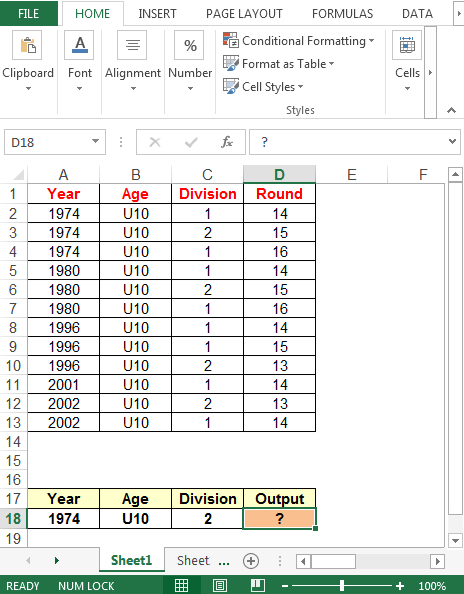We will use a combination of MAX & IF functions to get the result.

• In cell D18, the formula is
• {=MAX(IF((A2:A13=A18)*(B2:B13=B18)*(C2:C13=C18),D2:D13))}

Note: This is an array formula; CTRL + SHIFT = ENTER keys must be pressed together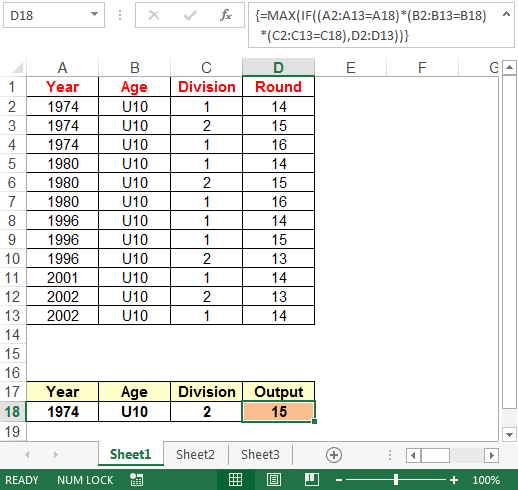Example 2

In second example, we want to get the max value meeting all the conditions.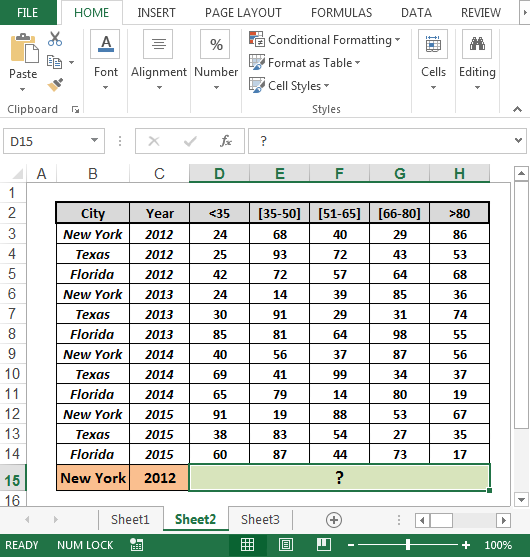This time we will use a combination of MAX & INDEX functions.

• In cell D15, the formula is
• =MAX(INDEX((B3:B14=B15)*(C3:C14=C15)*
D3:H14,0),0)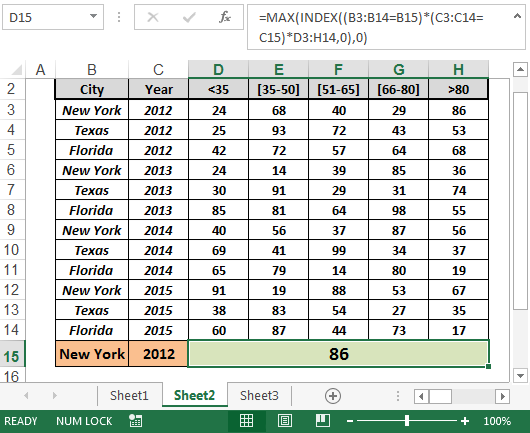In this way we can find out the maximum value, meeting the specific conditions.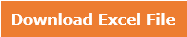Download - Formula to find MAX value based on multiple criteria - xlsx

Terms and Conditions of use

The applications/code on this site are distributed as is and without warranties or liability. In no event shall the owner of the copyrights, or the authors of the applications/code be liable for any loss of profit, any problems or any damage resulting from the use or evaluation of the applications/code.